PHY294H - Lecture21

The Hall effect

The Hall effect is a very important effect in solid state physics, particularly in semiconductor physics. It enables us to determine, amongst many other things, whether the dominant carriers are electrons or holes. It works by applying perpendicular electric and magnetic fields to a material which has a finite resistance. The electric field produces a current flow in the direction of the electric field, that is V=IR. The magnetic field initially leads to a bending of the charges which carry the current. However the bending of the current leads to a charge build up on the edges of the sample. This in turn leads to a voltage perpendicular to the direction of applied electric field and also perpendicular to the applied magnetic field. This is called the Hall voltage. Eventually, the Hall field is sufficient to balance the magnetic force on the current, so that,

 qEH = qvD B (1)

We thus find,(2)

Note that the polarity of the hall voltage has opposite signs for hole carriers and electron carriers.

The force between two current carrying wires

A DC current produces a magnetic field. This was observed by Oersted in 1819 and by Ampere in 1820. Ampere did a series of experiments to deduce the magnetic field near a long straight wire carrying a current I. He did this by studying the force between two current carrying wires. Consider two long straight wires which are parallel to each other and which carrying currents I1 and I2. Let us take the length of the wires to be L and their separation to be d. Ampere's experiments involved measuring the force between the two long wires. He found that the force is attractive for two wires which carry current in the same direction, while it is repulsive for wires carrying currents in opposite directions. The magnitude of the force was found to be,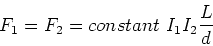(3)

The constant is defined to(4)

This is a rather annoying but intrenched piece of notation, the permeability is denoted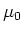, which should not be confused with the magnetic moment.sets the scale of forces and magnetic fields in magneto-statics in the same way that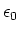(or k) sets the scale of forces and fields in electrostatics..

Now we want to deduce the magnitude and direction of the magnetic fields which are produced by the wires. Since a DC current produces a magnetic field, we may consider one of the wires to produce a magnetic field and the other wire to be influenced by it, that is,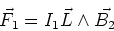(5)

In a similar way,(6)

By Newton's third law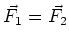. Comparing Eq. (3) and (5) it is evident that the magnitude of the magnetic field due to wire 1 is,(7)

Similarly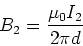(8)

Of course these magnetic fields are present even if an isolated wire carries a current, I, so we have the relation,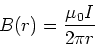(9)

which describes the magnetic field as a function of distance from the wire. The direction of the magnetic field must be perpendicular to the current and to the force between the wires. This indicates that the magnetic field circulates around the wire. The circulation of the magnetic field is given by the right hand rule, again.

Ampere realised that his result for the magnetic field near a wire may be written in the form of a path integral.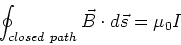(10)

Here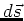is a small vector along the direction of the path. For example for a circle the unit vector is in tangent to the circle. In this case, we have,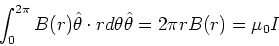(11)

Solving for B(r) yields equation (9). Eq. (10) is Ampere's law and is one of two tools that we will use to find the magnetic field produced by currents. In this form Ampere's law is correct only for DC, time independent currents. Later in the course we will add an additional term which enables us to use this equation even when there are time dependent fields.